# Third law of thermodynamics

The third law of thermodynamics (or Nernst's theorem after the experimental work of Walther Nernst in 1906 ) states that the entropy of a system approaches a minimum (that of its ground state) as one approaches the temperature of absolute zero. One can write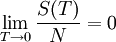$\lim_{T \rightarrow 0} \frac{S(T)}{N} = 0$

where$N$ is the number of particles. Note that there are systems whose ground state entropy is not zero, for example metastable states or glasses, or systems with weakly or non-coupled spins that are not subject to an ordering field.

## Implications

The heat capacity (for either pressure or volume) tends to zero as one approaches absolute zero. From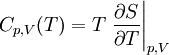$C_{p,V}(T)= T \left. \frac{\partial S}{\partial T} \right\vert_{p,V}$

one has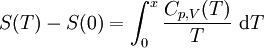$S(T) - S(0) = \int_0^x \frac{C_{p,V}(T)}{T} ~\mathrm{d}T$

thus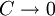$C \rightarrow 0$ as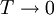$T \rightarrow 0$, otherwise the integrand would become infinite.

Similarly for the thermal expansion coefficient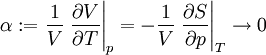$\alpha := \frac{1}{V} \left. \frac{\partial V}{\partial T} \right\vert_p = -\frac{1}{V} \left. \frac{\partial S}{\partial p} \right\vert_T \rightarrow 0$